Illustrations of Formulas
 What are Illustrations of  formulas? Simple Formulas Binomial Formulas Pythagoras's Theorem  Cubes References
 To the Main Page   "Mathematische Basteleien"

What are Illustrations of  formulas?

Formulas are statements of algebra, which apply to numbers of a definition set.

They can be proved except the axioms. You can deduce new formulas by well-known formulas by logical reasoning. This procedure is called a proof.

The proof  ideas and also the proof ways can be described by pictures. In addition the formulas themselves become more alive.

Simple Formulas  top

Commutative law of multiplication (Axiom)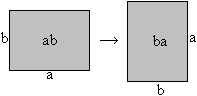ab=ba

Distributive law (Axiom)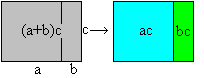(a+b)c=ac+bc

Product of a difference and a number(a-b)c=ac-bc

Product of two sums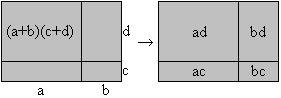Product of two differences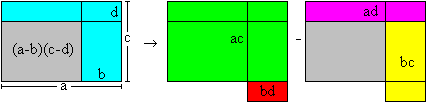Product of a sum and a differenceLooking for a parallelogram with the same areaa²=bx

Binomial Formulas   top

First binomial formula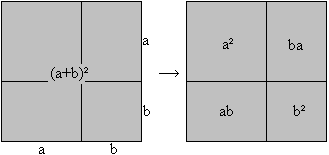(a+b)²=a² + 2ab + b²

Second binomial formula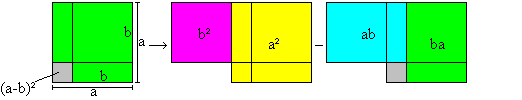(a-b)²=  b²+a²  -2ab

Third binomial formula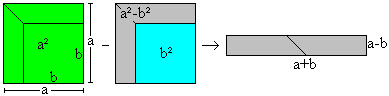a²-b²=(a+b)(a-b)

Tri-nomial formula(a+b+c)²=a²+b²+c²+2ab+2ac+2bc

Difference of the squares of a sum and a difference(a+b)²-(a-b)²=4ab

Pythagoras's Theorem  top

The Pythagorean theorem (Pythagoras or one of his students, Pythagoras of Samos, 580-500 BC)a²+b²=c²

The Pythagorean theorem (Euklid, ~300 BC)
Classical proof with triangles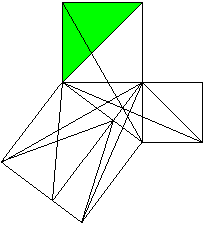a²+b²=c²

The Pythagorean theorem (Euklid, ~300 BC)
Proof with four-sided figuresa²+b²=c²

Euklid's theorem (Euklid, ~300 BC)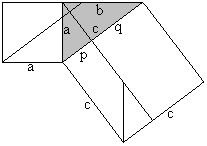a²=cp (You can show b²=cq in analogy.)

Height formula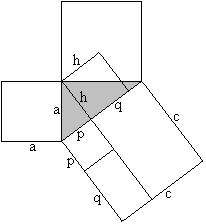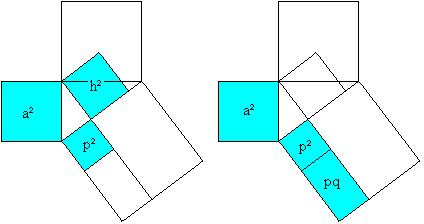a²=p²+h² (Pythagorean theorem), a²=pc=p²+pq (Euklid's theorem),
hence h²=pq

The Pythagorean theorem (Liu Hui, ~300, China)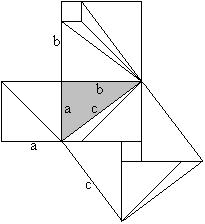a²+b²=c²

The Pythagorean theorem ("The bride's chair", ~900, India)a²+b²=c²

The Pythagorean theorem (Atscharja Bhaskara, Indien,  ~1150)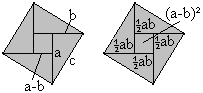c²=(a-b)²+2ab oder c²=a²+b²

The Pythagorean theorem (Leonardo da Vinci, 1452-1519)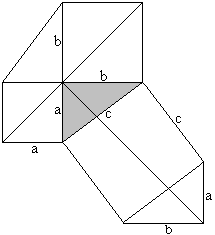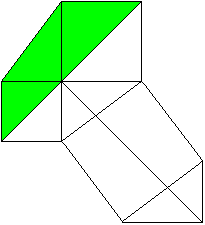a²+b²=c²

The Pythagorean theorem (Arthur Schopenhauer's case was a=b, 1788-1860)a²+b²=c²

The Pythagorean theorem (James Garfield 1876, later on the 20th US President)You use the formula of the area of a trapezium [A=mh, here h=a+b and m=(a+b)/2]
(a+b)²/2=c²/2+2*(1/2*ab) or a²+b²=c²

The Pythagorean theorem (Hermann Baravalle 1945)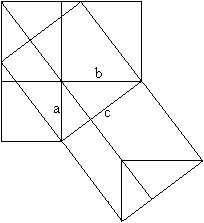.........c²=a²+b²

The Pythagorean theorem(a+b)²=c²+4*(1/2ab) oder a²+b²=c²

Cubes     top

Cube of a sum(a+b)³=a³+3a²b+3ab²+b³

You can see both cubes and the six rectangular parallelepipeds in 3D-view:Cube of a difference

The formula is (a-b)³=a³-3a²b+3ab²-b³. You convert it to (a-b)³=a³-3ab(a-b)-b³ for an illustration.
 ...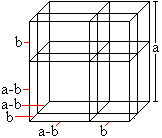... You take the drawing of the formula (a+b)³=a³+3a²b+3ab²+b³ from above and replace a by the difference a-b.  Then the edges are (a-b)+b with different combinations (on the left). The term (a-b)³ is illustrated by the blue cube (on the right). ......
You  recieve the blue cube, too, if you take away the three green bodies and the yellow cube from the red cube:(a-b)³ = a³-3ab(a-b)-b³ = a³-3a²b+3ab²-b³

References    top
Alexander Bogomolny
Martin Gardner, Mathematisches Labyrinth, Vieweg Braunschweig 1979 (ISBN 3-528-08402-2)
Johannes Lehmann (Hrsg.): Rechnen und Raten, Köln 1987 (ISBN 3-7614-0930-3)
A.Schmid, I. Weidig: Lambacher Schweizer S8, Mathematisches Unterrichtswerk, Stuttgart 1995 (ISBN 3-12-730730-6)
A.Schmid, I. Weidig: Lambacher Schweizer S9, Mathematisches Unterrichtswerk, Stuttgart 1996 (ISBN 3-12-730740-3)
Peter Baptist: Pythagoras und kein Ende? Leipzig, 1998 (ISBN 3-12-720040-4)

Feedback: Email address on my main page